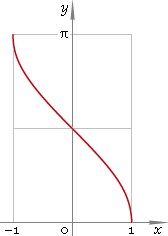The Art of Interface

# arccos — trigonometric arc cosine function

Category. Mathematics.

Abstract. Trigonometric arc cosine: definition, plot, properties, identities and table of values for some arguments.

## 1. Definition

Arc cosine is inverse of the cosine function.

## 2. Plot

Arc cosine is monotone function defined in the range [−1, 1]. Its plot is depicted below in fig. 1.Fig. 1. Plot of the arc cosine function y = arccosx.

Function codomain is limited to the range [0, π].

## 3. Identities

Complementary angle:

arcsinx + arccosx = π/2

and as consequence:

arccos sin φ = π/2 − φ

Negative argument:

arccos(−x) = π − arccosx

Reciprocal argument:

arcos(1/x) = arcsecx

Sum and difference:

arccosx + arccosy = arccos{xy − √[(1 − x2)(1 − y2)]}
arccosx − arccosy = arccos{xy + √[(1 − x2)(1 − y2)]}

Some argument values:

Argument xValue arccosx
0π/2
(√6 − √2) /45π/12
(√5 − 1) /42π/5
√(2 − √2) /23π/8
1 /2π/3
√(10 - 2√5) /43π/10
1 /√2π/4
(√5 + 1) /4π/5
√3 /2π/6
√(2 + √2) /2π/8
√(10 + 2√5) /4π/10
(√6 + √2) /4π/12
10
Table 1. Arc cosine for some argument values.

## 4. Support

Trigonometric arc cosine function arccos of the real argument is supported by free version of the Librow calculator.

Trigonometric arc cosine function arccos of the complex argument is supported by professional version of the Librow calculator.

## 5. How to use

To calculate arc cosine of the number:

``arccos(-1);``

To calculate arc cosine of the current result:

``arccos(rslt);``

To calculate arc cosine of the number x in memory:

``arccos(mem[x]);``